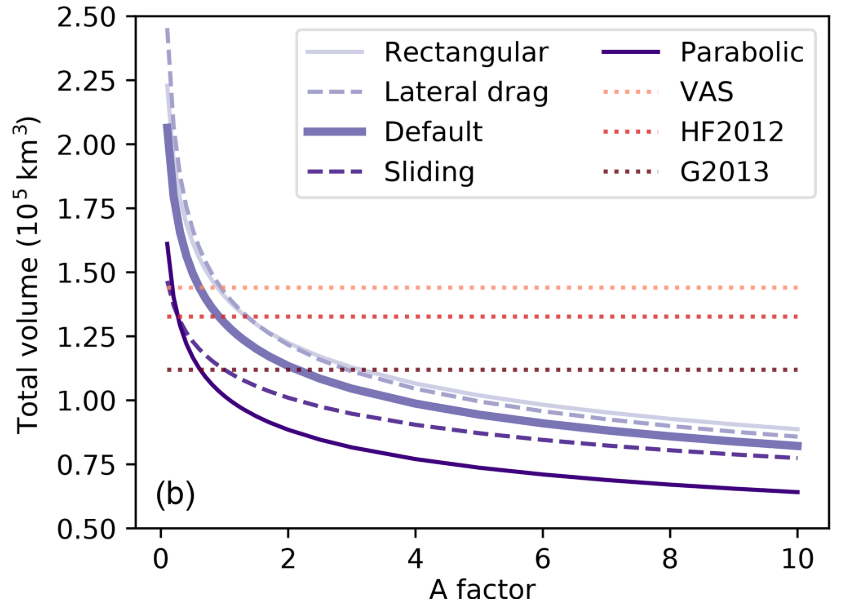# Pitfalls and limitations#

As the OGGM project is gaining visibility and momentum, we also see an increase of potential misuse or misunderstandings about what OGGM can and cannot do. Refer to our FAQ and Troubleshooting for a general introduction. Here, we discuss specific pitfalls in more details.

## The default ice dynamics parameter “Glen A” is roughly calibrated#

Out-of-the box, non-preprocessed OGGM will use fixed values for the creep parameter $$A$$ and the sliding parameter $$f_s$$:

In : from oggm import cfg

In : cfg.initialize()

In : cfg.PARAMS['glen_a']
Out: 2.4e-24

In : cfg.PARAMS['fs']
Out: 0.0


That is, $$A$$ is set to the standard value for temperate ice as given in [Cuffey_Paterson_2010], and sliding is set to zero. While these values are reasonable, they are unlikely to be the ones yielding the best results at the global scale, and even more unlikely at regional or local scales. In particular, in the absence of sliding parameter, it is recommended to set $$A$$ to a higher value to compensate for this missing process (effectively making ice “less stiff”).

New in version 1.4!

Since v1.4, OGGM can now calibrate $$A$$ based on the consensus from [Farinotti_etal_2019] on any number of glaciers. We recommend to use a large number of glaciers: OGGM’s default glacier directories are calibrated at the RGI region level. This value is then also used by the forward dynamical model for consistency, according to the parameter use_inversion_params_for_run.

The pre-processed directories at level 3 to 5 are already calibrated to the consensus estimate at the RGI region level, i.e. unless specified otherwise, OGGM will use the pre-calibrated $$A$$ value for these glaciers.

There is a way to calibrate $$A$$ for the ice thickness inversion procedure based on observations of ice thickness (see this blog post about g2ti for an example). At the global scale, a value in the range of [1.1-1.5] times the default value gives volume estimates close to [Farinotti_etal_2019]. At regional scale, these values can differ, with a value closer to a factor 3, for example for the Alps. Note that this depends on other variables as well, such as our estimates of solid precipitation amounts (i.e mass turnover). This makes things complicated, as regions with overestimated solid precipitation can be compensated by a higher $$A$$, and the other way around.

Finally, note that a change in $$A$$ has a very strong influence for values close to the default value, but this influence reduces to the power of 1/5 for large values of A (in other words, there is a big difference between values of 1 to 1.3 times the default $$A$$, but a comparatively small difference for values between 3 to 5 times the default $$A$$). This is best shown by this figure from [Maussion_etal_2019]:Global volume estimated as a function of the multiplication factor applied to the ice creep parameter A, with five different setups: defaults, with sliding velocity, with lateral drag, and with rectangular and parabolic bed shapes only (instead of the default mixed parabolic/rectangular). In addition, we plotted the estimates from standard volume–area scaling (VAS, $$V = 0.034 S^{1.375}$$), Huss and Farinotti (2012) (HF2012) and Grinsted (2013) (G2013). The latter two estimates are provided for indication only as they are based on a different glacier inventory#

Now, what you are probably asking yourself: how to choose the “best A” for my application?

Sorry, but we don’t know yet. We are working on it though! At the moment, what we recommend to do is to calibrate $$A$$ so that the regional (or even local) estimates match the volume consensus of Farinotti et al. (2019) using the workflow.calibrate_inversion_from_consensus() global task. This is what we do for the default pre-processed directories at the RGI region level, so that you don’t have to worry about it.

## The numerical model in OGGM is numerically unstable in some conditions#

OGGM uses a CFL criterion to decide on the timestep to use during the ice dynamics model iteration. The numerical scheme of OGGM is fast and flexible (e.g. it allows to compute the ice flow on multiple flowlines), but it is not following textbook recommendations on numerical stability.

See this github issue for a discussion pointing this out, and this example.

As of OGGM v1.2, we have fixed the most pressing issues. This blog post explains it in detail, but for a summary:

• the old algorithm was flawed, but did not result in significant errors at large scales

• the new algorithm is faster and more likely to be stable

• we don’t guarantee statibility in 100% of the cases, but when the model becomes unstable it will raise an error.

We test OGGM for mass-conservation in several use cases. What might happen, however, is that the calculated velocities display “wobbles” or artifacts, which are a sign of instability. If this occurs, set the global parameter cfg.PARAMS['cfl_number'] to a lower value (0.01 or 0.005 are worth a try).